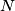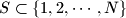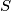MEMO 2012 pojedinačno problem 2

Kvaliteta:
Avg: 2,0
Težina:
Avg: 5,0
Let$N$ be a positive integer. A set$S \subset \{ 1, 2, \cdots, N \}$ is called allowed if it does not contain three distinct elements$a, b, c$ such that$a$ divides$b$ and$b$ divides$c$. Determine the largest possible number of elements in an allowed set$S$.
Izvor: Srednjoeuropska matematička olimpijada 2012, pojedinačno natjecanje, problem 2